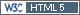## CVwR ~ CVwT ~ CVw? [Power / Sample Size]

Hello zizou,

You lost me.
I am sure your point may be good but I don't understand it. Could you reformulate these parts:

» Theoretically I can imagine (no real) data of 2x2x4 replicate study - see following idea:
» After Test treatment we will have almost the same values for each individual subject (e.g. subject No. 1 value 0.9501 after the first T and 0.9502 after the second T (before ln-transformation)) - for simplicity geometric mean of T values close to 0.95.
» In the same way after Reference treatment we will have almost the same values for each individual subject (e.g. subject No. 1 value (1/0.9501) after the first R and (1/0.9502) after the second R (before ln-transformation) - If all the values of R are reciprocal values of T we should get the same intra-subject CV for T and R.) - for simplicity geometric mean of R values equal to (1/0.95).

I am lost here.

» So GMR T/R of all "pooled" data will be close to 0.95/(1/0.95)=0.95^2=0.9025 and CVw used for CI calculation will be higher than CVwT and CVwR?

Does that follow automatically? How? Why? CVw used for CI calculation is a dangerous term when we talk EMA?
I tried to look at Helmut's simulated data and could still not figure it out.» I know, in this kind of example the CVwT and CVwR are extremely low, but the point is that theoretically the CVw observed in 2x2 study can be higher than 30% (so we can try replicate design with possible widening of BE acceptance criteria) but the CVwT and CVwR (which we don't know from 2x2) can be lower.

Imagine an old production process for R and a new process for T. This is real life.
Unit-to-unit variability for T is low, for R it is high. When you do a replicated study you see the effect directly on sWR, sWT. It is completely obscured for a 222BE trial. Hence it is hardly surprising that you'll be seeing little difference here and there between the RMSE from a 222BE trial and then the ones derived from the EMA method where replication is involved.

``` if (3) 4 x=c("Foo", "Bar") b=data.frame(x) typeof(b[,1]) ##aha, integer? b[,1]+1 ##then let me add 1 ```

Best regards,
ElMaestro

"(...) targeted cancer therapies will benefit fewer than 2 percent of the cancer patients they’re aimed at. That reality is often lost on consumers, who are being fed a steady diet of winning anecdotes about miracle cures." New York Times (ed.), June 9, 2018.Ing. Helmut Schütz